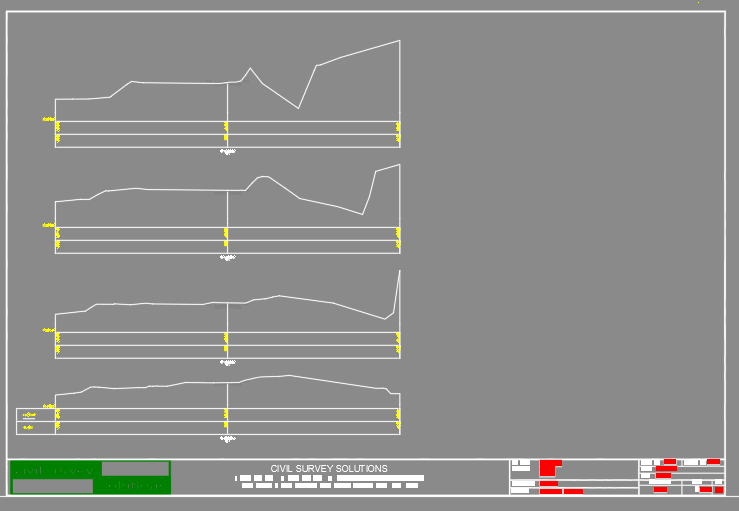# Cross_ Section.

## Recommended PostsHi friends i m new  in lisp.

i have regularly required cross section profile for  mines activity. any one can help me for cross section or 3d line profile. pls pls pls help me .

for more details i had attached my file.

pls pls pls help me.

##### Share on other sitesThis was CIV3D and Civil site design, 100m wide cross section 6 minutes and some 40 cross sections produced. just a case of changing some limits etc could be to full limit of surface.

If you have Briscad or Autocad then www.civilsurveysolutions.com.au will do so much more. But yes at a price.Edited by BIGAL

##### Share on other sitesHere is a basic solution:

```(defun KGA_List_LastRemove (lst)
(reverse (cdr (reverse lst)))
)

(defun c:CreateCrossSection ( / doc end enm fac lin lst ss sta vec)
(vla-endundomark doc)
(vla-startundomark doc)
(setvar 'cmdecho 0)
(if
(and
(= 1 (getvar 'worlducs))
(equal '(0.0 0.0 1.0) (getvar 'viewdir))
(setq lin (car (entsel "\nSelect line for cross section: ")))
(setq vec (getpoint "\nInsertion point for section: "))
(setq fac (getreal "\nHeight factor: "))
(progn (command "_.zoom" "_object" lin "") T)
(setq lin (entget lin))
(= "LINE" (cdr (assoc 0 lin)))
(setq sta (KGA_List_LastRemove (cdr (assoc 10 lin))))
(setq end (KGA_List_LastRemove (cdr (assoc 11 lin))))
(setq ss (ssget "_F" (list sta end) '((0 . "POLYLINE") (-4 . "&=") (70 . 8))))
(progn (command "_.zoom" "_previous") T)
)
(progn
(setq lst
(vl-remove
nil
(mapcar
'(lambda (itm)
(if
(and (listp itm) (= 0 (car itm)))
)
)
(apply 'append (ssnamex ss))
)
)
)
;; Sort points:
(setq lst
(vl-sort
lst
'(lambda (a b) (< (car a) (car b)))
)
)
;; Remove duplicate points:
(setq lst
(vl-remove
nil
(mapcar
'(lambda (cur prev)
(if (equal cur prev 1e-8) nil cur)
)
lst
(cons nil lst)
)
)
)
;; Calculate point for LW poly:
(setq vec (mapcar '* '(1.0 1.0 0.0) vec))
(setq lst
(mapcar
'(lambda (pt)
(list (+ (car pt) (car vec)) (+ (* fac (cadr pt)) (cadr vec)))
)
lst
)
)
;; Create LW poly:
(vlax-invoke (vla-get-modelspace doc) 'addlightweightpolyline (apply 'append lst))
;; Create base line:
(vlax-invoke (vla-get-modelspace doc) 'addline vec (mapcar '+ vec (list (distance sta end) 0.0 0.0)))
(command "_.zoom" "_extents")
)
)
(setvar 'cmdecho 1)
(vla-endundomark doc)
(princ)
)```

##### Share on other sitesRoy, your code is probably fine in BricsCAD, but when I test it under A2018, my (ssnamex ss) is returning wrong Z coordinate of ssget-fence intersection points... I don't know if this is the case and with others who have A2018 (I recently reinstalled it, so maybe that process bugged my CAD), but nevertheless I found solution to this particular case...

I changed this :

```      (setq lst
(vl-remove
nil
(mapcar
'(lambda (itm)
(if
(and (listp itm) (= 0 (car itm)))
)
)
(apply 'append (ssnamex ss))
)
)
)```

To this :

```      (setq lst
(vl-remove
nil
(mapcar
'(lambda (itm)
(if
(and (listp itm) (= 0 (car itm)))
(list (distance sta (KGA_List_LastRemove (cadr itm))) (caddr (vlax-curve-getclosestpointtoprojection (vl-some '(lambda (x) (if (= (type x) 'ename) x)) (vl-member-if '(lambda (x) (equal x itm)) (reverse (apply 'append (ssnamex ss))))) (KGA_List_LastRemove (cadr itm)) '(0.0 0.0 1.0))))
)
)
(apply 'append (ssnamex ss))
)
)
)```

And it seems that this mod. fixed the problem...

I only don't know why is now my CAD buggy... Can someone verify this issue with OP's posted DWG and AutoCAD testing?

Does someone had this problem and was the solution found? (z coordinates are always with the same value although it's obvious that they should differ...)

Edited by marko_ribar

##### Share on other sitesI did respond as its a CIVIL Engineering question, so the freebie answer is here at Cadtutor its a triangulation solution by YMG just search I think it has had cross sections added. There are some others out there also just google Triangulation & Lisp. I have been involved in this type of thing since Green screen PC's.

Roy_043 I would not spend the time it is reinventing the wheel. I produced  40 sheets with 3 cross sections per sheet it takes about 15 seconds to change parameters like Hor and Ver scales and a regen reproduces the sheets.

The dwg provided appears to have been produced in CIV3D so why not cross sections produced ? It would have been far more accurate to have started with a triangulation which probably exists but was not in dwg.

.

Edited by BIGAL

##### Share on other sites@marko_ribar Thanks for looking at my code. The original code indeed works in BricsCAD (V18). I typically test my code before posting.

## Join the conversation

You can post now and register later. If you have an account, sign in now to post with your account.
Note: Your post will require moderator approval before it will be visible.×   Pasted as rich text.   Paste as plain text instead

Only 75 emoji are allowed.